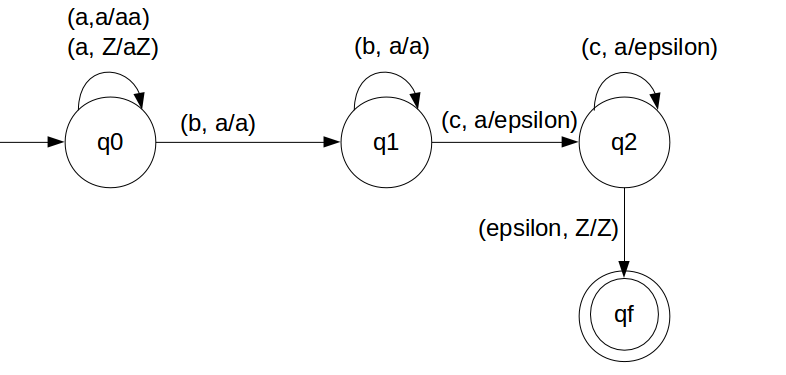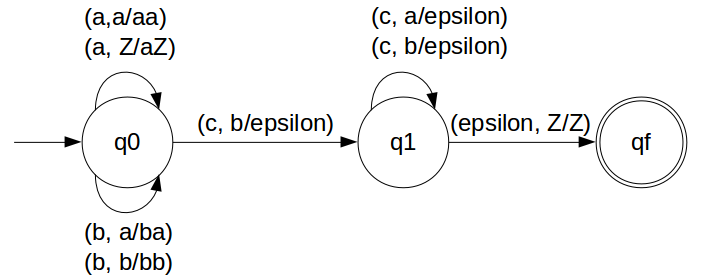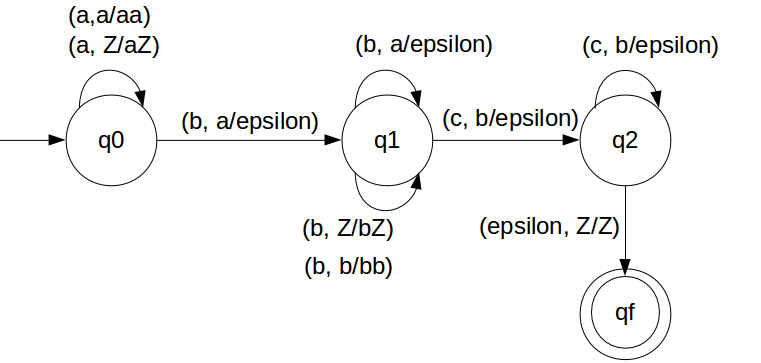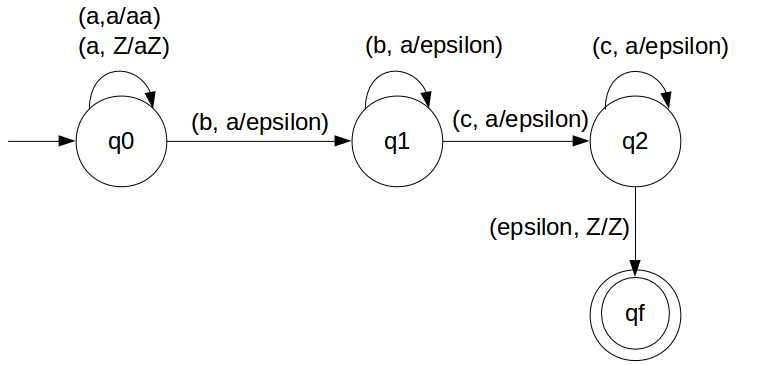DPDA for anbmcn n,m≥1

Approch is quite similar to previous example, we just need to look for bm.
First we have to count number of a's and that number should be equal to number of c's.
That we will achieve by pushing a's in STACK and then we will pop a's whenever "c" comes.
But we have to take care b's coming between 'a' and 'c'.
For occurance of b's we will do nothing, means we will not make in changes in STACK for b's.

So in the end of the strings if nothing is left in the STACK then we can say that language is accepted in the PDA.

We have designed the PDA for the problem:### STACK Transiton Function

```		δ(q0, a, Z) = (q0, aZ)
δ(q0, a, a) = (q0, aa)

δ(q0, b, a) = (q1, a)
δ(q1, b, a) = (q1, a)

δ(q1, c, a) = (q2, ε)
δ(q2, c, a) = (q2, ε)

δ(q2, ε, Z) = (qf, Z)
```

Note: qf is Final State

### Explanation

Lets see, how this DPDA is working:
We will take one input string: "aabbbcc"
1. Scan string from left to right
2. First input is 'a' and follow the rule:
3. on input 'a' and STACK alphabet Z, push the input 'a' into STACK as : (a,Z/aZ) and state will be q0
4. Second input is 'a' and so follow the rule:
5. on input 'a' and STACK alphabet 'a', push the input 'a' into STACK as : (a,a/aa) and state will be q0
6. Third input is 'b' and so follow the rule:
7. on input 'b' and STACK alphabet 'a', do nothing as : (b,a/&epsiloon;) and state will be now q1
8. Fourth input is 'b' and so follow the rule:
9. on input 'b' and STACK alphabet 'a', do nothing as : (b,a/&epsiloon;) and state will remain q1
10. Fifth input is 'b' and so follow the rule:
11. on input 'b' and STACK alphabet 'a', do nothing as : (b,a/&epsiloon;) and state will remain q1
12. Sixth input is 'c' and top of STACK is 'a' so follow the rule:
13. on input 'c' and STACK alphabet 'a' and state is q1, pop one 'a' as : (c,a/ε) and state will be q2
14. Seventh input is 'c' and top of STACK is 'a' so follow the rule:
15. on input 'c' and STACK alphabet 'a' and state is q2, pop one 'a' as : (c,a/ε) and state will remain q2
16. We reached end of the string, so follow the rule:
17. on input ε and STACK alphabet Z, go to final state(qf) as : (ε, Z/Z)

The explanation might be looking over descriptive but please be patient, It will all get clear once you start to get along with the explanation.
So we suggest you to keep doing the practice with the steps mentioned in the explnation

DPDA for anbmc(n+m) n,m≥1

Just see the given problem in another perspective.
As add number of a's and b's, and that will equal to number of c's.
So for every a's and b's we will pop c's from the STACK.
First we have to count number of a's and b's that total number should be equal to number of c's.
That we will achieve by pushing a's and b's in STACK and then we will pop a's and b's whenever "c" comes.

So in the end of the strings if nothing is left in the STACK then we can say that language is accepted in the PDA.

We have designed the PDA for the problem:### STACK Transiton Function

```		δ(q0, a, Z) = (q0, aZ)
δ(q0, a, a) = (q0, aa)
δ(q0, b, a) = (q0, ba)
δ(q0, b, b) = (q0, bb)

δ(q0, c, b) = (q1, ε)
δ(q1, c, b) = (q1, ε)
δ(q1, c, a) = (q1, ε)

δ(q1, ε, Z) = (qf, Z)
```

Note: qf is Final State

### Explanation

Lets see, how this DPDA is working:
We will take one input string: "aabbcccc"
1. Scan string from left to right
2. First input is 'a' and follow the rule:
3. on input 'a' and STACK alphabet Z, push the input 'a' into STACK as : (a,Z/aZ) and state will be q0
4. Second input is 'a' and so follow the rule:
5. on input 'a' and STACK alphabet 'a', push the input 'a' into STACK as : (a,a/aa) and state will be q0
6. Third input is 'b' and so follow the rule:
7. on input 'b' and STACK alphabet 'a', push the input 'b' into STACK as : (b,a/ba) and state will be q0
8. Fourth input is 'b' and so follow the rule:
9. on input 'b' and STACK alphabet 'b', push the input 'b' into STACK as : (b,b/bb) and state will be q0
10. Fifth input is 'c' and so follow the rule:
11. on input 'c' and STACK alphabet 'b' and state is q0, pop one 'b' as : (c,b/ε) and state will be q1
12. Sixth input is 'c' and top of STACK is 'b' so follow the rule:
13. on input 'c' and STACK alphabet 'b' and state is q1, pop one 'b' as : (c,b/ε) and state remain q1
14. Seventh input is 'c' and top of STACK is 'a' so follow the rule:
15. on input 'c' and STACK alphabet 'a' and state is q1, pop one 'a' as : (c,a/ε) and state will be q1
16. Eighth input is 'c' and top of STACK is 'a' so follow the rule:
17. on input 'c' and STACK alphabet 'a' and state is q1, pop one 'a' as : (c,a/ε) and state will be remain q1
18. We reached end of the string, so follow the rule:
19. on input ε and STACK alphabet Z, go to final state(qf) as : (ε, Z/Z)

The explanation might be looking over descriptive but please be patient, It will all get clear once you start to get along with the explanation.
So we suggest you to keep doing the practice with the steps mentioned in the explnation

DPDA for anb(n+m)cm n,m≥1

Just see the given problem in another perspective. As:

DPDA for anbnbmcn n,m≥1

So we will design the DPDA for the approach we have suggested.

### Clarification

First we have to count number of a's and that number should be equal to number of b's
When all a's are finished by b's
Secondly count number of b's and that should be equal to number of c's
So in the end of the strings if nothing is left in the STACK then we can say that language is accepted in the PDA.

We have designed the PDA for the problem:### STACK Transiton Function

```		δ(q0, a, Z) = (q0, aZ)
δ(q0, a, a) = (q0, aa)
δ(q0, b, a) = (q1, ε)
δ(q1, b, a) = (q1, ε)

δ(q1, b, Z) = (q1, bZ)
δ(q1, b, b) = (q1, bb)
δ(q1, c, b) = (q2, ε)
δ(q2, c, b) = (q2, ε)

δ(q2, ε, Z) = (qf, Z)
```

Note: qf is Final State

### Explanation

Lets see, how this DPDA is working:
We will take one input string: "aabbbbcc"
1. Scan string from left to right
2. First input is 'a' and follow the rule:
3. on input 'a' and STACK alphabet Z, push the input 'a' into STACK as : (a,Z/aZ) and state will be q0
4. Second input is 'a' and so follow the rule:
5. on input 'a' and STACK alphabet 'a', push the input 'a' into STACK as : (a,a/aa) and state will be q0
6. Third input is 'b' and so follow the rule:
7. on input 'b' and STACK alphabet 'a', pop one 'a' from STACK as : (b,a/ε) and state will be q1
8. Fourth input is 'b' and so follow the rule:
9. on input 'b' and STACK alphabet 'a' and state q1, pop one 'a' from STACK as : (b,a/ε) and state will remain q1
10. Fifth input is 'b' and so follow the rule:
11. on input 'b' and STACK alphabet 'Z' and state is q1, push one 'b' as : (b,Z/bZ) and state will remain q1
12. Sixth input is 'b' and so follow the rule:
13. on input 'b' and STACK alphabet 'b' and state is q1, push one 'b' as : (b,b/bb) and state will remain q1
14. Seventh input is 'c' and top of STACK is 'b' so follow the rule:
15. on input 'c' and STACK alphabet 'a' and state is q1, pop one 'b' as : (c,b/ε) and state will be q2
16. Eighth input is 'c' and top of STACK is 'b' so follow the rule:
17. on input 'c' and STACK alphabet 'b' and state is q2, pop one 'b' as : (c,b/ε) and state will be remain q2
18. We reached end of the string, so follow the rule:
19. on input ε and STACK alphabet Z, go to final state(qf) as : (ε, Z/Z)

The explanation might be looking over descriptive but please be patient, It will all get clear once you start to get along with the explanation.
So we suggest you to keep doing the practice with the steps mentioned in the explnation

DPDA for a(n+m)bmcn n,m≥1

Just see the given problem in another perspective.
As add number of b's and c's, and that will equal to number of a's.
So for every b's and c's we will pop a's from the STACK.
First we have to count number of a's and that number should be equal to number of b's and c's.
That we will achieve by pushing a's in STACK and then we will pop a's whenever "b"/"c" comes.

So in the end of the strings if nothing is left in the STACK then we can say that language is accepted in the PDA.

We have designed the PDA for the problem:### STACK Transiton Function

```		δ(q0, a, Z) = (q0, aZ)
δ(q0, a, a) = (q0, aa)
δ(q0, b, a) = (q1, ε)
δ(q1, b, a) = (q1, ε)
δ(q1, c, a) = (q2, ε)
δ(q2, c, a) = (q2, ε)

δ(q2, ε, Z) = (qf, Z)
```

Note: qf is Final State

### Explanation

Lets see, how this DPDA is working:
We will take one input string: "aaaabbcc"
1. Scan string from left to right
2. First input is 'a' and follow the rule:
3. on input 'a' and STACK alphabet Z, push the input 'a' into STACK as : (a,Z/aZ) and state will be q0
4. Second input is 'a' and so follow the rule:
5. on input 'a' and STACK alphabet 'a', push the input 'a' into STACK as : (a,a/aa) and state will be q0
6. Third input is 'a' and so follow the rule:
7. on input 'a' and STACK alphabet 'a', push the input 'a' into STACK as : (a,a/aa) and state will be q0
8. Fourth input is 'a' and so follow the rule:
9. on input 'a' and STACK alphabet 'a', push the input 'a' into STACK as : (a,a/aa) and state will be q0
10. Fifth input is 'b' and so follow the rule:
11. on input 'b' and STACK alphabet 'a' and state is q0, pop one 'a' as : (c,a/ε) and state will be q1
12. Sixth input is 'b' and top of STACK is 'a' so follow the rule:
13. on input 'b' and STACK alphabet 'a' and state is q1, pop one 'a' as : (c,a/ε) and state remain q1
14. Seventh input is 'c' and top of STACK is 'a' so follow the rule:
15. on input 'c' and STACK alphabet 'a' and state is q1, pop one 'a' as : (c,a/ε) and state will be q2
16. Eighth input is 'c' and top of STACK is 'a' so follow the rule:
17. on input 'c' and STACK alphabet 'a' and state is q2, pop one 'a' as : (c,a/ε) and state will be remain q2
18. We reached end of the string, so follow the rule:
19. on input ε and STACK alphabet Z, go to final state(qf) as : (ε, Z/Z)